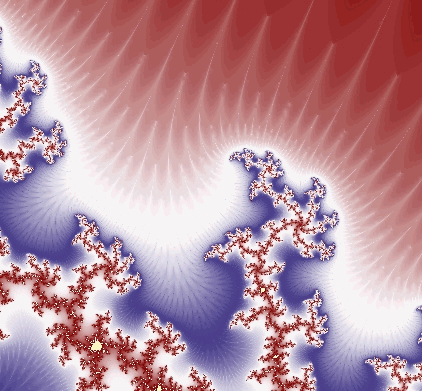Search IntMath
Close

450+ Math Lessons written by Math Professors and Teachers

5 Million+ Students Helped Each Year

1200+ Articles Written by Math Educators and Enthusiasts

Simplifying and Teaching Math for Over 23 Years

# Fractal Science Kit

By Murray Bourne, 29 Jun 2008

I heard from the creator of Fractal Science Kit during the week.

He wrote:

I have just released the Fractal Science Kit 1.0 and thought you might be interested in taking a look. The Fractal Science Kit provides an interactive programming environment with windows for viewing the fractal image, modifying the properties that define the fractal, examining the data behind the fractal, and viewing/editing the programs, macros (inline functions/methods), and color gradients, used by the Fractal Science Kit to produce the final image.

The programming language you use to develop your programs, supports a complete set of control structures including if statements, while loops, for loops, switch statements, inline functions/methods, arrays, and user defined objects. The complex data type is the fundamental variable type, and arithmetic operators and functions handle complex operands/arguments. A rich set of built-in functions/methods are included, and you can develop your own library of functions/methods for use throughout the application.

During the development of the application, I developed 100s of sample programs to illustrate how the application can be used to generate many different types of fractals including: Mandelbrot, Julia, Convergent, Newton, Orbit Traps, Sierpinski Triangle, IFS, Strange Attractors, Rep-N Tiles, Symmetric Icons, Apollonian Gasket, Circle Inversion, Schottky Group, Kleinian Group, L-System and many more.

For additional details visit Fractal Science Kit. The web site contains a complete description of the product and provides the full documentation, galleries, and tutorials.

I had a play with the software and found it to be more sophisticated than the other fractal generators I have come across. Here's one of the first fractals I created. I love the swirls of white.For those who are not sure what fractals have got to do with mathematics, check out Fractals and complex numbers.

That link again: Fractal Science Kit

Be the first to comment below.

### Comment Preview

HTML: You can use simple tags like <b>, <a href="...">, etc.

To enter math, you can can either:

1. Use simple calculator-like input in the following format (surround your math in backticks, or qq on tablet or phone):
a^2 = sqrt(b^2 + c^2)
(See more on ASCIIMath syntax); or
2. Use simple LaTeX in the following format. Surround your math with $$ and $$.
$$\int g dx = \sqrt{\frac{a}{b}}$$
(This is standard simple LaTeX.)

NOTE: You can mix both types of math entry in your comment.